• Openpyxl教程（2020年9月2日） 开始了。 第一步：安装环境 python环境（anaconda继承环境更傻瓜式一点） 安装openpyxl conda install openpyxl 第二步：了解excel的基本环境 行（row）列（column）格子（cell...
Openpyxl教程（2020年9月2日）
开始了。
第一步：安装环境
python环境（anaconda继承环境更傻瓜式一点）  安装openpyxl conda install openpyxl
第二步：了解excel的基本环境
行（row）列（column）格子（cell）工作表（sheet）
第三步：
基本操作
进入 jupyter环境
juoyter notebook
快速补齐快捷键–Tab
打开Excel表格并获取表格名称
from openpyxl import load_workbook
workbook.sheetnames
通过sheets名获得内容
sheet1 = workbook["Sheet1"]
print(sheet1)
）获取表格的尺寸大小 （几行列数据 几行列数据 )
sheet1.dimensions
获取表格内某个格子的数据
方法1：
sheet2 = workbook.active
print(sheet2)
cell1 = sheet2["A2"]
print(cell1.value)

方法2：
cell1 = sheet.cell(row = 1,column = 1)
获取某个变量的值，行，列，sheet，坐标
print(cell1.value, cell1.row, cell1.column, cell1.coordinate)
print(cell2.value, cell2.row, cell2.column, cell2.coordinate)
获取多个格子的数据
# 获取A1:C2区域的值
cell = sheet["A1:H12"]
print(cell)
for i in cell:
for j in i:
print(j.value)
sheet["A"] --- 获取A列的数据
sheet["A:C"] --- 获取A,B,C三列的数据
sheet --- 只获取第5行的数据

按行读取和按列读取
# 按行获取值
for i in sheet2.iter_rows(min_row=2, max_row=5, min_col=1, max_col=2):
for j in i:
print(j.value)
# 按列获取值
for i in sheet2.iter_cols(min_row=2, max_row=5, min_col=1, max_col=2):
for j in i:
print(j.value)
#查询所有的表格
for i in sheet2.rows():
print(i.value)
向EXCEL写入数据
功能1：修改表格中的内容
#方法1
sheet1["A1"] = "hello world"
#方法2
cellA1 = sheet1["A1"]
cellA1.value = "hello world"

""" 注意：我们将“A1”单元格的数据改为了“哈喽”，
并另存为了“哈喽.xlsx”文件。
如果我们保存的时候，不修改表名，相当于直接修改源文件； """

功能2：.append()向表格中插入行数据 (很有用 )
注意回顾一下 tuple（元组，不可更改的集合），list（有序，可重复集合），set（无序，不可重复集合），dist（字典，键值对）
#新建tuple---（）小括号
tuple1 = （”a“，”b“，”c“）
tuple2 = tuple(list1)
tuple3 = tuple("hello world")
#新建list----[]中括号
list1 = [”a“，”b“，”c“]

#新建set,必须由set函数生成或者{}----{}大括号
set1 = set([”a“，”b“，”c“])
set2 = {”a“，”b“，”c“}

#新建字典dist
#创建一个空字典
empty_dict = dict()
print(empty_dict)

#用**kwargs可变参数传入关键字创建字典
a = dict(one=1,two=2,three=3)
print(a)

#传入可迭代对象
b = dict(zip(['one','two','three'],[1,2,3]))
print(list(zip(['one','two','three'],[1,2,3])))
print(b)

#传入可迭代对象
c = dict([('one', 1), ('two', 2), ('three', 3)])
print(c)

c1 = dict([('one', 1), ('two', 2), ('three', 3),('three', 4),('three', 5)])
print(c1)#如果键有重复，其值为最后重复项的值。
#传入映射对象，字典创建字典
d = dict({'one': 1, 'two': 2, 'three': 3}) 
功能3：使用excel函数公式（***很重要***）
for i in range(2,16):
sheet["D{}".format(i)] = 			'=IF(RIGHT(C{},2)="cm",C{},SUBSTITUTE(C{},"m","")*100&"cm")'.format(i,i,i)
EXCEL里常用的公式
frozenset({'ISLOGICAL', 'YIELDDISC', 'DAYS360', 'CUBEKPIMEMBER', 'IMSUB', 'MATCH', 'CUBEMEMBER', 'IMSUM', 'ODDFYIELD', 'CUBEMEMBERPROPERTY', 'TRUNC', 'IMEXP', 'DSTDEV', 'CUBEVALUE', 'COS', 'COMBIN', 'SECOND', 'SQRTPI', 'COSH', 'ISNONTEXT', 'MODE', 'QUARTILE', 'DPRODUCT', 'DATEVALUE', 'NORMSDIST', 'DMIN', 'HARMEAN', 'ISERROR', 'LOGINV', 'AVERAGEIFS', 'GAMMALN', 'BETADIST', 'DGET', 'TODAY', 'NEGBINOMDIST', 'ABS', 'AND', 'LN', 'SIGN', 'MAX', 'SUMX2MY2', 'SEARCHB', 'HYPGEOMDIST', 'RIGHTB', 'ODDLPRICE', 'PERCENTRANK', 'DAY', 'RAND', 'DOLLAR', 'BETAINV', 'PHONETIC', 'ODDLYIELD', 'BINOMDIST', 'EDATE', 'TEXT', 'GCD', 'EXP', 'COUPDAYS', 'TBILLYIELD', 'CRITBINOM', 'YIELDMAT', 'DAVERAGE', 'IRR', 'PV', 'SIN', 'RANK', 'NETWORKDAYS', 'ATAN2', 'MROUND', 'GROWTH', 'INFO', 'DSTDEVP', 'COUNTIF', 'LOGNORMDIST', 'FV', 'CUBERANKEDMEMBER', 'PROB', 'IMREAL', 'HEX2BIN', 'SUMXMY2', 'ISNUMBER', 'IMABS', 'GETPIVOTDATA', 'BIN2DEC', 'NOMINAL', 'COUNTBLANK', 'ROMAN', 'MIRR', 'AREAS', 'GESTEP', 'SUMX2PY2', 'DMAX', 'JIS', 'PRICEMAT', 'TTEST', 'DELTA', 'SUBSTITUTE', 'COLUMN', 'EFFECT', 'REPLACE', 'QUOTIENT', 'STDEVP', 'ATANH', 'TIMEVALUE', 'RADIANS', 'BESSELK', 'COUNT', 'PMT', 'IFERROR', 'MDETERM', 'GAMMAINV', 'CELL', 'LEFT', 'NETWORKDAYS.INTL', 'CUBESET', 'MULTINOMIAL', 'DB', 'VARPA', 'IF', 'FINDB', 'CONVERT', 'DURATION', 'MINUTE', 'SYD', 'COUPPCD', 'MIN', 'VAR', 'TRIM', 'DEC2OCT', 'OFFSET', 'ERF', 'RANDBETWEEN', 'MINA', 'FLOOR', 'HLOOKUP', 'AVERAGE', 'VDB', 'IMPOWER', 'DDB', 'RIGHT', 'LOGEST', 'PROPER', 'SINH', 'CHOOSE', 'MDURATION', 'INTRATE', 'PPMT', 'LCM', 'INT', 'NORMDIST', 'LOWER', 'MINVERSE', 'N', 'SMALL', 'REPLACEB', 'CONFIDENCE', 'IMCOS', 'STDEV STDEVA', 'ECMA.CEILING', 'ASINH', 'FISHERINV', 'OCT2DEC', 'INDEX', 'SUMIF', 'AVERAGEIF', 'AMORLINC', 'CORREL', 'ERFC', 'FISHER', 'OCT2HEX', 'TINV', 'PERCENTILE', 'BIN2OCT', 'ISREF', 'WEEKDAY', 'POWER', 'XIRR', 'TAN', 'COUPDAYSNC', 'RECEIVED', 'ACCRINT', 'T', 'KURT', 'MEDIAN', 'IMLOG10', 'POISSON', 'LEFTB', 'LOOKUP', 'COLUMNS', 'INTERCEPT', 'ERROR.TYPE', 'EVEN', 'IMCONJUGATE', 'CEILING', 'VLOOKUP', 'TBILLPRICE', 'IMAGINARY', 'PRICE', 'FREQUENCY', 'CHAR', 'NPV', 'VARP', 'IMARGUMENT', 'COMPLEX', 'SUMIFS', 'ROW', 'HEX2OCT', 'WORKDAY ', 'PRICEDISC', 'CHIINV', 'DATE', 'NOT', 'PI', 'DOLLARDE', 'RATE', 'AMORDEGRC', 'SLOPE', 'ACOSH', 'FIND', 'BESSELY', 'WEIBULL', 'NORMINV', 'ODD', 'IMSQRT', 'TANH', 'SERIESSUM', 'ATAN', 'COUNTA', 'FORECAST', 'FIXED', 'TIME', 'XNPV', 'NOW', 'FDIST', 'VALUE', 'BIN2HEX', 'PRODUCT', 'IMSIN', 'CHITEST', 'STANDARDIZE', 'SKEW', 'SUMSQ', 'COUPNCD', 'MMULT', 'LENB', 'COUNTIFS', 'DEVSQ', 'DSUM', 'EOMONTH', 'ISPMT', 'BESSELJ', 'TRIMMEAN', 'DATEDIF', 'PERMUT', 'ODDFPRICE', 'LOG10', 'VARA', 'EXACT', 'INDIRECT', 'LEN', 'SUMPRODUCT', 'SUBTOTAL', 'DOLLARFR', 'ISEVEN', 'DEC2BIN', 'ISO.CEILING', 'WORKDAY.INTL', 'DISC', 'LARGE', 'RTD', 'FALSE', 'FINV', 'YEAR', 'IMPRODUCT', 'IPMT', 'REPT', 'SUM', 'DEGREES', 'MIDB', 'UPPER', 'MOD', 'ROUNDDOWN', 'CODE', 'DEC2HEX', 'MAXA', 'CUBESETCOUNT', 'COVAR', 'FACTDOUBLE', 'HEX2DEC', 'GEOMEAN', 'ISTEXT', 'ACCRINTM', 'RSQ', 'ISNA', 'AVEDEV', 'LOG', 'TDIST', 'WEEKNUM', 'CLEAN', 'IMLN', 'CHIDIST', 'FTEST', 'PEARSON', 'SQRT', 'DCOUNTA', 'NPER', 'AVERAGEA', 'YEARFRAC', 'OCT2BIN', 'MONTH', 'ZTEST', 'STDEVPA STEYX', 'FACT', 'ASIN', 'IMLOG2', 'SLN', 'BAHTTEXT', 'ROWS', 'SEARCH', 'YIELD', 'COUPDAYBS', 'ASC', 'ACOS', 'FVSCHEDULE', 'ISBLANK', 'TBILLEQ', 'TRUE ADDRESS', 'MID', 'HYPERLINK', 'CUMPRINC', 'DVARP', 'DCOUNT', 'NA', 'TRANSPOSE', 'IMDIV', 'ISERR', 'OR', 'ISODD', 'CUMIPMT', 'EXPONDIST', 'TREND', 'GAMMADIST', 'LINEST', 'HOUR', 'ROUND', 'TYPE', 'BESSELI', 'ROUNDUP', 'CONCATENATE', 'COUPNUM', 'DVAR', 'NORMSINV'})

插入行和插入列
sheet2.insert_col(idx = "数字编号",amount="要插入的列数")
sheet2.insert_rows(idx= "数字编号"，amount="要插入的行数")
删除行和列
* .delete_rows(idx=数字编号, amount=要删除的行数)
* .delete_cols(idx=数字编号, amount=要删除的列数)
移动格子
#move_range()
sheet1.move_range("C2:D4",rows=2,cols=-1)
创建新的sheet表格
workbook.create_sheet("新建一个sheet")
删除某个sheet表
workbook.remove(sheet1)
把sheet复制到另一张excel里去
workbook.copy_worksheet(sheet1)
修改sheet名
sheet1.title = "新的的sheet名"
创建新的 excel 表格文件 表
from openpyxl import Workbook
workbook = Workbook()
sheet = workbook.active
sheet.title = "表格1"
workbook.save(filename = "新建的excel表格")
.freeze_panes：冻结窗口
这个也不常用，只是看到了，顺便提一下
下面就比较经典了
给表格添加筛选器
workbook = load_workbook(filename = "花园.xlsx")
sheet = workbook.active
print(sheet)
sheet.auto_filter.ref = sheet["A1"]
workbook.save(filename = "花园.xlsx")
批量修改字体样式
cell = sheet["A1"]
font = Font(name="微软雅黑",size=20,bold=True,italic=True,color="FF0000")
cell.font = font
workbook.save(filename = "花园.xlsx")
获取表格中格子的字体样式
font = cell.font
设置对齐样式
Alignment(horizontal=水平对齐模式,vertical=垂直对齐模式,text_rotation=旋转角度,wrap_text=是否自动换行)水平对齐：‘distributed’，‘justify’，‘center’，‘leftfill’， ‘centerContinuous’，‘right，‘general’；垂直对齐：‘bottom’，‘distributed’，‘justify’，‘center’，‘top’；
cell.alignment = alignment
设置边框样式
Side(style=边线样式，color=边线颜色)Border(left=左边线样式，right=右边线样式，top=上边线样式，bottom=下边线样式)style参数的种类： 'double, ‘mediumDashDotDot’, ‘slantDashDot’, ‘dashDotDot’,‘dotted’,‘hair’, 'mediumDashed, ‘dashed’, ‘dashDot’, ‘thin’, ‘mediumDashDot’,‘medium’, ‘thick’
side1 = Side(style="thin",color="FF0000")
side2 = Side(style="thick",color="FFFF0000")
border = Border(left=side1,right=side1,top=side2,bottom=side2)
cell.border = border
设置填充样式
PatternFill（fill_type）
设置行高和列宽
sheet = workbook.active
# 设置第1行的高度
sheet.row_dimensions.height = 50
# 设置B列的宽度
sheet.column_dimensions["B"].width = 20
合并单元格
sheet.merge_cells("C1:D2")
sheet.merge_cells(start_row=7,start_column=1,end_row=8,end_column=3)

* .unmerge_cells(待合并的格子编号)
* .unmerge_cells(start_row=起始行号，start_column=起始列号，end_row=结束行号，end_column=结束列号)
展开全文python
• 有小伙伴反映自己在一些文章中看到openpyxl也能对Excel进行相关的操作，于是留言想在本公众号里也能看到相关的教程。于是我开始了本专题的写作。另外，在推文《操作Excel的Xlwings教程(一)》中，我对比了几种操作...在以前的推文中，我们介绍了操作Excel的模块Xlwings的知识，相关推文可以从本公众号的底部相关菜单获取。有小伙伴反映自己在一些文章中看到openpyxl也能对Excel进行相关的操作，于是留言想在本公众号里也能看到相关的教程。于是我开始了本专题的写作。另外，在推文《操作Excel的Xlwings教程(一)》中，我对比了几种操作Excel的模块，大家可以去了解一下。
1.openpyxl简介
openpyxl是用于读取/写入Excel 2010 xlsx/xlsm文件的Python库，也就是说openpyxl这个Python库不支持xls文件的读取和操作，如果在工作中遇到xls文件我们就不能使用这个库。官方说它的诞生是因为缺少可从Python本地读取/写入Office Open XML格式的库，为了方便大家就开发了这个库，这是非常棒的。
2.文件转换
上述提到openpyxl只能操作xlsx文件，当我们遇到xls文件的时候就需要进行转化，转换方式这里提供几种方案供大家参考：
方法一：手动打开xlsx文件，然后另存为xlsx类型的文件。
方法二：使用pywin32模块进行转换，示例代码如下：
import os
import win32com.client as win32
filename = r'C:\Users\XH\Desktop\1.xls'
Excelapp = win32.gencache.EnsureDispatch('Excel.Application')
workbook = Excelapp.Workbooks.Open(filename)
# 转xlsx时: FileFormat=51,
# 转xls时:  FileFormat=56,
workbook.SaveAs(filename.replace('xls', 'xlsx'), FileFormat=51)
workbook.Close()
Excelapp.Application.Quit()
# 删除源文件
# os.remove(filename)

# 如果想将xlsx的文件转换为xls的话，则可以使用以下的代码：
# workbook.SaveAs(filename.replace('xlsx', 'xls'), FileFormat=56)


方法三：使用pandas模块进行转换，代码如下：
import pandas as pd
filename = r'C:\Users\XH\Desktop\1.xls'
filename2 = r'C:\Users\XH\Desktop\1.xlsx'
read_res.to_excel(filename2, index=False)
方法三在很多情况下出现一定的错误，比如在很多时候因为源表格的问题会造成数据丢失类的错误。个人推荐使用第二种方法。
3.基本操作-创建工作簿
安装openpyxl这个模块非常简单，cmd窗口中输入: pip install openpyxl。无需在文件系统上创建文件即可开始使用openpyxl。接下来我们来进行一个简单操作：
1、创建一个新的名为Mytest.xlsx文件。
2、在工作簿上第一个位置新建一个名为“mytest”的sheet页。
我们可以这样来实现，代码中每一个操作上都有相应的注释：
from openpyxl import Workbook
# 创建一个工作簿对象
wb = Workbook()
# 在索引为0的位置创建一个名为mytest的sheet页
ws = wb.create_sheet('mytest',0)
# 对sheet页设置一个颜色（16位的RGB颜色）
ws.sheet_properties.tabColor = 'ff72BA'
# 将创建的工作簿保存为Mytest.xlsx
wb.save('Mytest.xlsx')
# 最后关闭文件
wb.close()
最后生成的文件样式如下：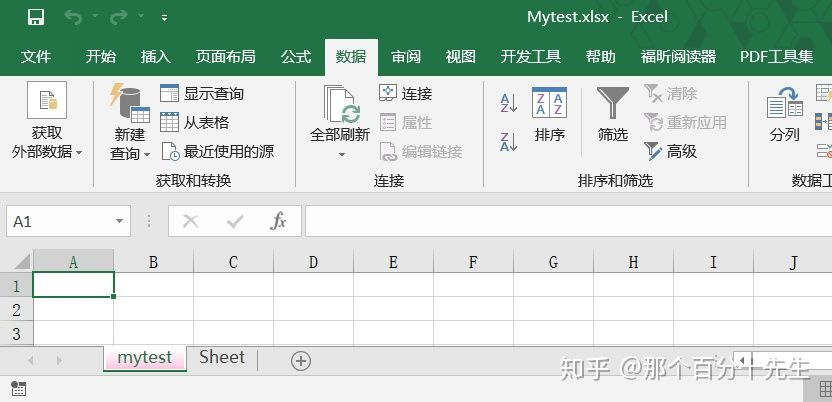那么打开已有的文件Mytest.xlsx，读取一些信息怎么操作呢?我们可以这样：
from openpyxl import load_workbook
# 加载工作簿
# 获取sheet页
ws2 = wb2['mytest']
ws3 = wb2.get_sheet_by_name('mytest')
# 打印sheet页的颜色属性值
print('color:',ws2.sheet_properties.tabColor)
wb2.close()
上述代码的输出如下，可以看到一些属性值或参数：
color: <openpyxl.styles.colors.Color object>
Parameters:
rgb='00ff72BA', indexed=None, auto=None, theme=None, tint=0.0, type='rgb'
另外，有些小伙伴可能看到上述代码中，ws2和ws3都是获取sheet页签的。的确这两种方法的效果是一样的，大家在平时的工作中都可以使用。
如果想获取这个工作簿的所有sheet页，可以这样：
print(wb2.sheetnames)
注意sheetnames属性值是一个列表，输出的结果为一个列表：['mytest', 'Sheet']使用for循环也是可以的：
for each_sheet in wb2.sheetnames:
print('each_sheet:',each_sheet)
4.基本操作-访问单元格
使openpyxl访问单元格很简单，分单个单元格访问和多个单元格的访问。我们接着来学习：访问单元格的方式一般也有两种做法：假设现在我们要访问单个单元格A1，我们可以这样：
cell_1 = ws2['A1']cell_2 = ws2.cell(row=1, column=1)
如果要取得这个单元格的内容，只需要在结尾加上value属性就可以了：
value_1 = ws2['A1'].value
value_2 = ws2.cell(row=1, column=1).value
如果需要给单元格进行设置值，则可以这样实现，比如给单元格A1设置内容：
ws2['A1'].value = 'python知识学堂'
ws2.cell(row=1, column=1).value ='python知识学堂'

对于多个单元格的设置就要借助for循环了。注意，设置后要保存工作簿，否则没有效果。
多个单元格的获取一般需要用到列表切片的知识或者使用for循环来进行:
# 访问A1至C3范围单元格
cell_range = ws2['A1':'C3']
# 访问A列所有存在数据的单元格
colA = ws2['A']
# 访问A列到C列所有存在数据的单元格
col_range = ws2['A:C']
# 访问第1行所有存在数据的单元格
row1 = ws2
# 访问第1行至第5行所有存在数据的单元格
row_range = ws2[1:5]

注意，上述cell_range等对象都是<class 'tuple'>类型的。如果先获取这些单元格中的值，我们可以这样：
for each_cell in cell_range:
for each in each_cell:
print(each.value)

for each_cell in colA:
print(each_cell.value)
至于为什么获取cell_range和colA的for循序的次数不一样，这个问题就留给大家自己了。

for循环的方式访问多个单元格可以这样：
for row in ws2.iter_rows(min_row=1, max_col=2, max_row=2):
for cell in row:
print(cell)
#输出：
<Cell 'mytest'.A1>
<Cell 'mytest'.B1>
<Cell 'mytest'.A2>
<Cell 'mytest'.B2>

for col in ws2.iter_cols(min_row=1, max_col=2, max_row=2):
for cell in col:
print(cell)
#输出：
<Cell 'mytest'.A1>
<Cell 'mytest'.A2>
<Cell 'mytest'.B1>
<Cell 'mytest'.B2>

这两个方法是按行优先和列优先的顺序进行访问单元格的。
大家可以新建一个工作簿，然后在工作簿中写入一些数据，之后运行以下代码看看打印一些什么：
print(tuple(ws2.rows))
print(tuple(ws2.columns))
注意：ws2.rows和ws2.columns是generator对象，因此需要使用tuple进行“解析”下。
5.最后的注意
使用openpyxl进行工作的时候，当一个工作结束的时候我们需要进行Excel文件的保存操作：wb.save('Mytest.xlsx')。这个保存唯一需要注意的是：文件是默认替换的。也就是说我们在保存文件的时候，openpyxl将进行替换而不发出告警。如果大家想保存不同阶段的文件，则可以在保存文件的时候加一个时间戳。
6.总结
以上就是本次的推文，推文介绍的内容比较简单，大家跟着学习的时候最好也跟着实践一下。后期我们将继续介绍其他方面的知识。比如在Excel中使用公式，合并单元格等操作。大家在学习的时候有什么疑问，也欢迎在评论区留言。
展开全文python excel
• 在之前的推文中，我们介绍了操作Excel的模块openpyxl的cell单元格操作，相关推文可以从本公众号的底部相关菜单获取。接下来的推文我们来学习一下openpyxl这个python模块中的其他知识，想了想还是先来学习一下怎么...在之前的推文中，我们介绍了操作Excel的模块openpyxl的cell单元格操作，相关推文可以从本公众号的底部相关菜单获取。接下来的推文我们来学习一下openpyxl这个python模块中的其他知识，想了想还是先来学习一下怎么借助openpyxl来进行Excel的公式设置。
一.公式数量和种类
我们先看一下在openpyxl中能使用的公式有哪些，我们来看代码：
from openpyxl.utils import FORMULAE
print(len(FORMULAE))
print(FORMULAE)
输出的内容如下：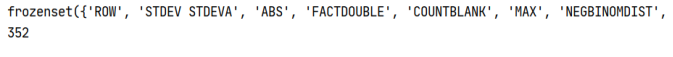可以看出支持的公式有ROW，ABS，MAX等等。支持的公式的数量可能因为版本不同而不一样，目前我使用的openpyxl的版本是3.0.5，支持公式的数量为352。
那么我们怎么判断自己要使用的公式是否支持，则可以简单的进行判断：
print('MID' in FORMULAE)
print('minddd' in FORMULAE)
上述代码输出True和False，也就是说公式“MID”支持，公式‘minddd’是不支持的。
二.在单元格中使用公式
假设我们现在有一个数据表“formulae_1.xlsx”，数据显示如下：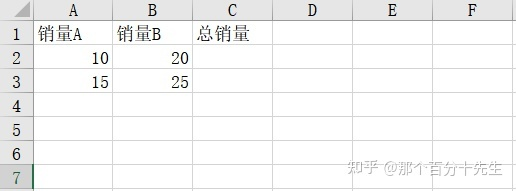我们将使用公式来计算一个总值，代码如下：
wbook = load_workbook(filename='formula_1.xlsx')
wsheet = wbook['Sheet1']
wsheet["C2"] = "=SUM(A2,B2)"
print(wsheet['C2'].value)
wbook.save("formula_1.xlsx")
代码中的输出为：=SUM(A2,B2)，操作后的表格显示如下，正好是两者之和。当然了上述第四行代码我们也可以这样写：wsheet["C2"] = "=SUM(10,20)"，也能得到我们下图的结果：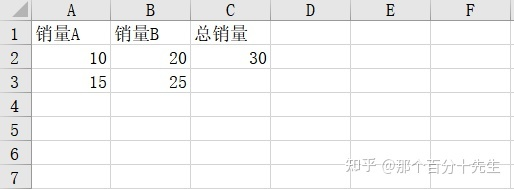利用for循环，我们可以将上述表格中的所有行的值：
from openpyxl import load_workbook
wsheet = wbook['Sheet1']
for j in range(2,4):
cell_a = 'A' + str(j)
cell_b = 'B' + str(j)
cell_c = 'C' + str(j)
wsheet[cell_c] = "=SUM({},{})".format(cell_a,cell_b)
wbook.save("formula_1.xlsx")
我们来看一下表格中的数据，选中单元格C2，可以看出这个单元格显示的是一个公式：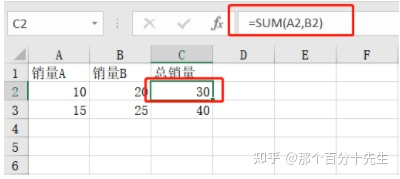那我们重新加载这个表格并读取 这个单元格会出现什么呢？
from openpyxl import load_workbook
wsheet = wbook['Sheet1']
cell_C2 = wsheet.cell(2,3).value
print(cell_C2)
wbook.save("formula_1.xlsx")
代码中的打印输出为：=SUM(A2,B2)。那么这里就存在一个问题，我们怎么得到这个单元格中计算的值呢？也就是如何打印出30。这里就要提及到openpyxl中的一个打开文件时的参数了，我们将第二行代码更改为：
wbook = load_workbook(filename='formula_1.xlsx',data_only=True)
我们运行替换后的代码的后，打印cell_C2值的时候是为却为None，这是为什么呢？查询相关的资料的解释可以参考：
https://blog.51cto.com/antidarkness/1974684
那么这个问题该怎么解决呢？我们可以使用win32库进行打开关闭的操作之后，在使用openpyxl打开文件传入data_only=True即可，使用win32打开保存的代码如下：（注意filaname需要传一个全路径）
from win32com.client import Dispatch
filename = r'C:\Users\LEGION\Desktop\tweets_code\formula_1.xlsx'
xlApp = Dispatch('Excel.Application')
xlApp.Visible = False
xlBook = xlApp.Workbooks.Open()
xlBook.Save()
xlBook.Close()
当然了在计算多行值的时候如A1到A5的和的时候，可使用："=SUM(A1:A5)"。
三.转化公式位置
将公式从一个位置转换到另一个位置在日常的工作中也是非常常见的，那么该怎么实现呢？我们先看一个代码：
from openpyxl import load_workbook
from openpyxl.formula.translate import Translator
wsheet = wbook['Sheet1']
wsheet['C3'] = Translator("=SUM(A2,B2)", origin="C2").translate_formula("C3")
print(wsheet['C3'].value)
wbook.save("formula_1.xlsx")
上述代码将"=SUM(A2,B2)"显示的公式，通过转变复制到C3单元格中了，print打印的结果为：=SUM(A3,B3)。C3单元格中的值也就变成了A3+B3的值了，是不是很有意思。官方文档对这个Translator(object)类的作用以及参数的解释为：

Modifies a formula so that it can be translated from one cell to another.

formula: The str string to translate. Must include the leading '=' character.

origin: The cell address (in A1 notation) where this formula was defined (excluding the worksheet name).

这里补充上次单元格中的一个知识点：
当我们在设置格式的时候没有自己想要的格式，那该怎么办呢？很简单，我们可以先使用Excel设置相应单元格的样式，然后使用下述代码打印格式：
cell_style = wsheet.cell(i,j).number_format
print(cell_style)
四.总结
以上就是本次的推文，推文介绍的单元格中使用公式的相关操作，大家跟着学习的时候最好也跟着实践一下。后期我们将继续介绍其他方面的知识。如进行表格的数据的筛选过滤等操作。大家在学习的时候有什么疑问，也欢迎在公众号后台留言。
展开全文python excel
• 接下来的推文我们来看一下在openpyxl中是怎么设置图表，或者说来看看图表中的相关知识。 一、图表种类 openpyxl中支持的图表种类比较丰富，有面积图、条形图、柱形图、散点图和饼状图等等，具体的图表种类显示...在上次的推文中，我们介绍了opnepyxl和numpy、pandas的结合使用的一些简单案例，相关推文可以从本公众号的底部相关菜单获取。接下来的推文我们来看一下在openpyxl中是怎么设置图表，或者说来看看图表中的相关知识。
一、图表种类
openpyxl中支持的图表种类比较丰富，有面积图、条形图、柱形图、散点图和饼状图等等，具体的图表种类显示如下：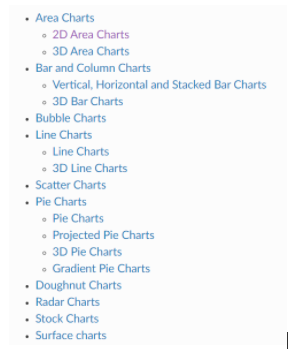这里列举了一些相关的图表种类，实际的工作中，我们可根据自己的需要进行图表的设置，一些相应的用法我们可以从在这个链接里找到答案，图表种类链接如下：
二、创建图表
我们先学习一下怎么简单的在Excel中创建一个树状图，我们先看代码：
from openpyxl import Workbook
from openpyxl.chart import BarChart, Reference, Series
workbook = Workbook()
worksheet = workbook.active
for i in range(10):
worksheet.append([i])
values = Reference(worksheet, min_col=1, min_row=1, max_col=1, max_row=10)
chart = BarChart()
workbook.save("TestChart.xlsx")
我们先看一下生成的"TestChart.xlsx"结果：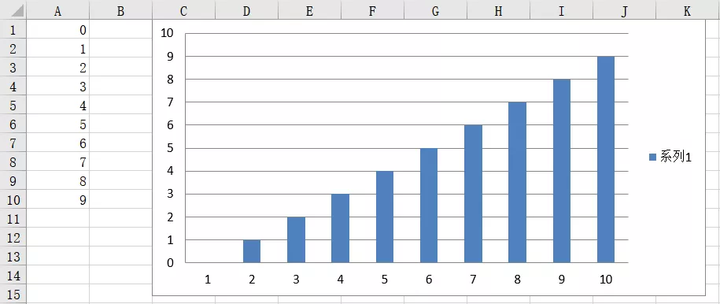代码解释：
第3-6行代码用于创建一个新的工作簿并写入0-10的数据。 第7行代码设置表格的数据范围，最大行为10。 第8行和第9行代码分别为新建一个chart对象，写入相应的数据。 第10行代码将表格添加到Excel中，“C1”表示图表在Excel中的左上角位置。
官方文档中介绍到：
上述的默认参数我们可以在“_char.py”的类CharBase()中找到相应的默认设置值，截
图如下：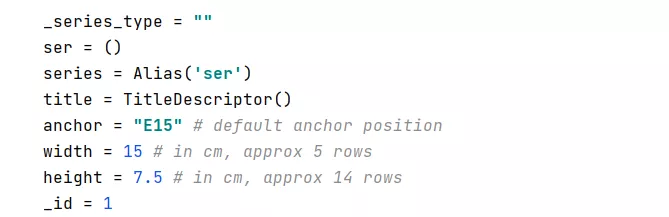也就是说我们可以自己设置图表的一些属性，比如我们更改上述一些代码如下：
values = Reference(worksheet, min_col=1, min_row=1, max_col=1, max_row=10)
chart = BarChart()
chart.anchor = 'C1'
chart.height = 5
chart.width = 10
workbook.save("TestChart.xlsx")
上述代码将图表的锚点设置在C1处，height为5，width为10。
三、横纵轴设置
openpyxl支持对图表的轴进行一些设置，比如我们可以手动设置轴的最大值和最小值。这样就可以以自定义的方式使得目标图表在图表上显示特定区域。这里我们直接以官方文档的例子来进行说明就好，先看一下代码：
设置轴
from openpyxl import Workbook
from openpyxl.chart import ScatterChart,Reference,Series
workbook = Workbook()
worksheet = workbook.active
worksheet.append(['X', '1/X'])
for x in range(-10, 11):
if x:
worksheet.append([x, 1.0 / x])
chart1 = ScatterChart()
chart1.title = "Full Axes"
chart1.x_axis.title = 'x'
chart1.y_axis.title = '1/x'
chart1.legend = None
chart2 = ScatterChart()
chart2.title = "Clipped Axes"
chart2.x_axis.title = 'x'
chart2.y_axis.title = '1/x'
chart2.legend = None
chart2.x_axis.scaling.min = 0
chart2.y_axis.scaling.min = 0
chart2.x_axis.scaling.max = 11
chart2.y_axis.scaling.max = 1.5
x = Reference(worksheet, min_col=1, min_row=2, max_row=22)
y = Reference(worksheet, min_col=2, min_row=2, max_row=22)
s = Series(y, xvalues=x)
chart1.append(s)
chart2.append(s)
workbook.save("minmax.xlsx")
代码解释：
首先使用append方法在Excel中增加了两列数据，然后添加了两个表，他们的表title分别为Full Axes和Clipped Axes。代码中使用的是x_axis.title和y_axis.title来进行坐标轴名称的设置的。在此之后使用了scaling.min和scaling.max进行一些坐标轴范围的设置，最后创建图表数据并添加到Excel中并设置图表的起始位置。下图为最后生成的表：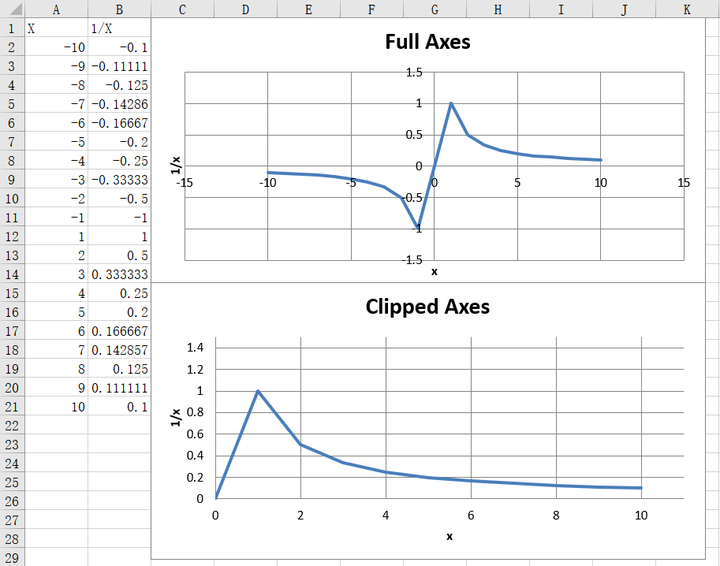官网提到我们还可以使用对数对图表的X轴和Y轴进行相应的缩放，即使用：
chart.x_axis.scaling.logBase = 10
chart.y_axis.scaling.logBase = 10
chart.x_axis.scaling.logBase = math.e
chart.y_axis.scaling.logBase = math.e
当然了轴的方向也是可以设置的，这个方向是使用orientation属性进行控制的，这个参数的值可选minMax和maxMin。在实际的使用过程中我们可以类似这样来进行设置：
chart.x_axis.scaling.orientation = "minMax"
chart.y_axis.scaling.orientation = "maxMin"
添加第二个轴
在实际的工程项目中，我们需要使用一个X坐标，却需要两个Y坐标，这时候添加第二个轴就很有用了，显然新创建的Y轴实际上涉及创建与第一个图表共享x轴但具有单独的y轴的第二个图表，我们来看一下代码实现：
from openpyxl import Workbook
from openpyxl.chart import LineChart,BarChart,Reference,Series
wb = Workbook()
ws = wb.active
rows = [
['sale_orange', 5, 6, 3, 9, 11, 7],
['sale_apple', 5, 7, 6, 10, 13, 5],
]
for row in rows:
ws.append(row)
# 创建第一个chart
c1 = BarChart()
v1 = Reference(ws, min_col=1, min_row=1, max_col=7)
c1.x_axis.title = 'Days'
c1.y_axis.title = 'Orange'
c1.y_axis.majorGridlines = None
c1.title = 'Sale Result'
# 创建第二个chart
c2 = LineChart()
v2 = Reference(ws, min_col=1, min_row=2, max_col=7)
c2.y_axis.axId = 0
c2.y_axis.title = "Apple"
# 将第二张图表的y轴设置为与x轴最大交叉
c1.y_axis.crosses = "max"
c1 += c2
wb.save("sale_result.xlsx")
结果如下，可以看出这个图画的还是相当不错的，实际中可以直接拿来使用！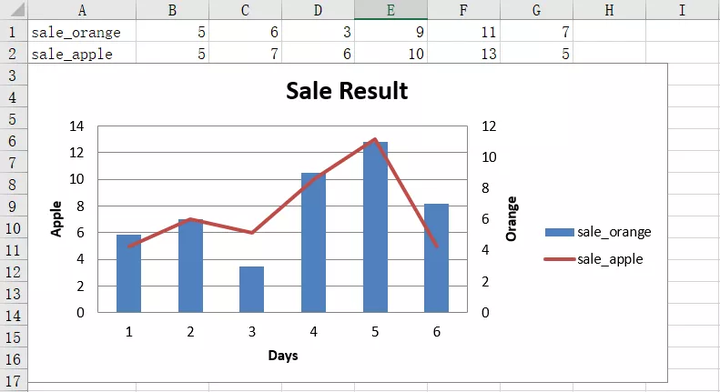四、图表布局设置
我们可以对图表的布局进行设置，使用x，y调整位置，w和h调整大小。 x是从左到右的水平距离，y是从顶部开始的垂距，h是高度，w是宽度。
图标中的图例也是可以设置的，图例的位置可以通过设置它的位置进行控制 r，l，t，b，和tr分别右，右，左，顶部，底部和顶部。openpyxl默认值为r。我们来看官网中的一个例子：
from copy import deepcopy
from openpyxl.chart import ScatterChart, Series, Reference
from openpyxl.chart.layout import Layout, ManualLayout
wb = Workbook()
ws = wb.active
rows = [
['Size', 'Batch_1', 'Batch_2'],
[2, 40, 30],
[3, 40, 25],
[4, 50, 30],
[5, 30, 25],
[6, 25, 35],
[7, 20, 40],
]
for row in rows:
ws.append(row)
ch1 = ScatterChart()
xvalues = Reference(ws, min_col=1, min_row=2, max_row=7)
for i in range(2, 4):
values = Reference(ws, min_col=i, min_row=1, max_row=7)
series = Series(values, xvalues, title_from_data=True)
ch1.series.append(series)
# 默认格式
ch1.title = "Default layout"
ch1.style = 13
ch1.x_axis.title = 'Size'
ch1.y_axis.title = 'Percentage'
ch1.legend.position = 'r'
# 一半大小，居于右下角
ch2 = deepcopy(ch1)
ch2.title = "Manual chart layout"
ch2.legend.position = "tr"
ch2.layout=Layout(
manualLayout=ManualLayout(
x=0.25, y=0.25,
h=0.5, w=0.5,
)
)

# 一半大小，居中
ch3 = deepcopy(ch1)
ch3.layout = Layout(
ManualLayout(
x=0.25, y=0.25,
h=0.5, w=0.5,
xMode="edge",
yMode="edge",
)
)
ch3.title = "Manual chart layout, edge mode"

# 手动将图例置于左下角
ch4 = deepcopy(ch1)
ch4.title = "Manual legend layout"
ch4.legend.layout = Layout(
manualLayout=ManualLayout(
yMode='edge',
xMode='edge',
x=0, y=0.9,
h=0.1, w=0.5
)
)
wb.save("chart_layout.xlsx")
Excel中生成的图表如下所示：在以后的需求中我们可以进行相应的设置。在layout.py中的类ManualLayout中可以找到XMode和YMode的相关设置。另外在openpyxl中整个数据系列和单个数据点可以设置一些属性。主要是使用openpyxl.drawing.fill中的一些方法：PatternFillProperties, ColorChoice。这里直接给出例子：
from openpyxl import Workbook
from openpyxl.chart import BarChart, Reference
from openpyxl.chart.marker import DataPoint
from openpyxl.drawing.fill import PatternFillProperties, ColorChoice
wb = Workbook()
ws = wb.active
rows = [
("Sample",),
(1,),
(2,),
(3,),
(2,),
(3,),
(3,),
(1,),
(2,),
]

for r in rows:
ws.append(r)
c = BarChart()
data = Reference(ws, min_col=1, min_row=1, max_row=9)
c.title = "Chart with patterns"
# 设置模式
series = c.series
fill =  PatternFillProperties(prst="pct5")
fill.foreground = ColorChoice(prstClr="red")
fill.background = ColorChoice(prstClr="blue")
# 使模式生效
series.graphicalProperties.pattFill = fill
# 对数据点进行设置，index默认从0开始
pt = DataPoint(idx=5)
pt.graphicalProperties.pattFill = PatternFillProperties(prst="ltVert")
series.dPt.append(pt)

wb.save("pattern.xlsx")
代码解释和说明：
prst="ltVert"是一种设置，还有其他值可以，如['pct5', 'pct10', 'pct20', 'pct25', 'pct30', 'pct40']等等，具体可以参考fill.py中的类PatternFillProperties()。 另外在官方文档中提及到一种Gauge Charts的图表，这里我就不在介绍了，大家可 以参考链接：https://openpyxl.readthedocs.io/en/stable/charts/gauge.html。
五、总结
以上就是本次的推文，推文介绍的是在Excel中进行排序的相关操作，大家跟着学习的时候最好也跟着实践一下。
展开全文python excel
• 在上期的推文中，我们介绍了使用openpyxl来进行公式的设置等操作，相关推文可以从本公众号的底部相关菜单获取。接下来的推文我们来学习一下openpyxl这个python模块中的其他知识，我们这次的推文来看一下怎么使用...python excel
• 在以前的文章中，我们介绍了操作Excel的模块openpyxl的入门知识，相关文章可以从主页获取。接下来我们来学习一下openpyxl这个python模块中的其他知识，本次文章我们来学习一下单元格（cell）操作的相关知识。 1....python 机器学习 人工智能
• 在上次的推文中，我们介绍了使用Openpyxl来进行数据的过滤和排序的操作， 相关推文可以从本公众号的底部相关菜单获取。在那篇推文中我们使用到了Pandas。因此本次推文我们就来看一看Openpyxl和Numpy、Pandas结合...python excel
• 接下来的推文我们来看一下在openpyxl中怎么对工作簿的Sheet进行一些设置。 一、工作表的可用属性 最常用的属性是“fitTopage”页面设置属性和tabColor，它们定义工作表选项卡的背景色。目前的openpyxl支持的可用...python...